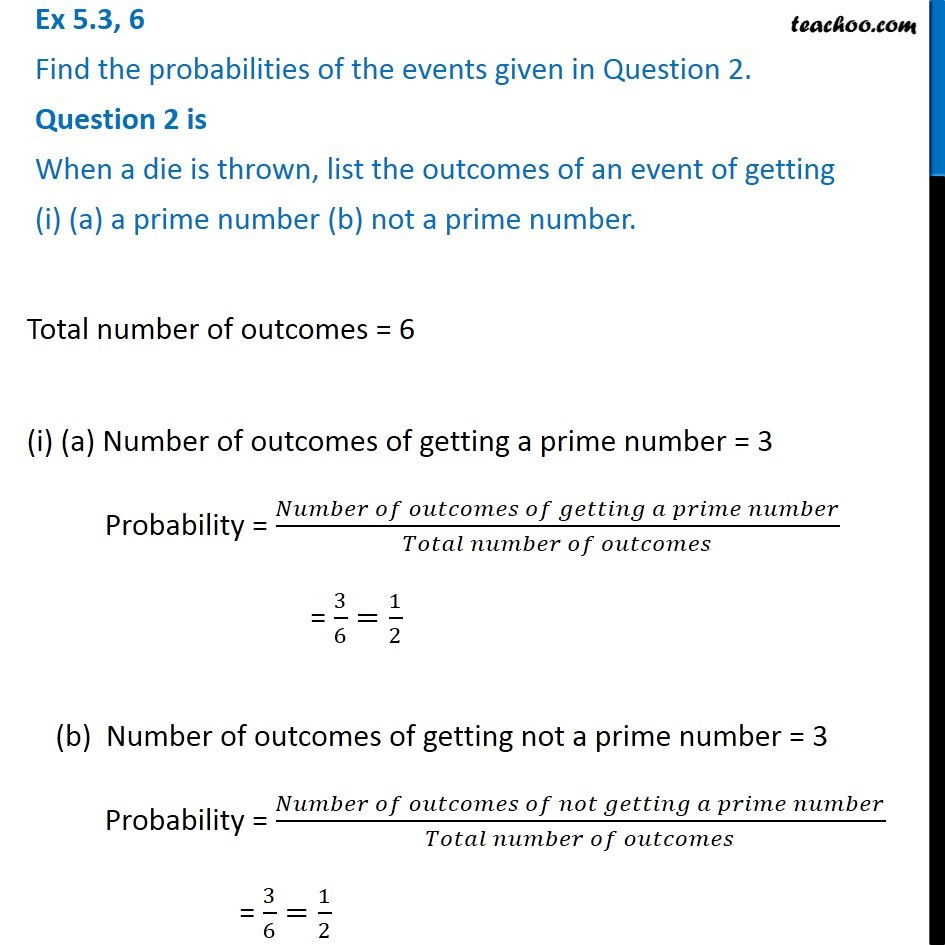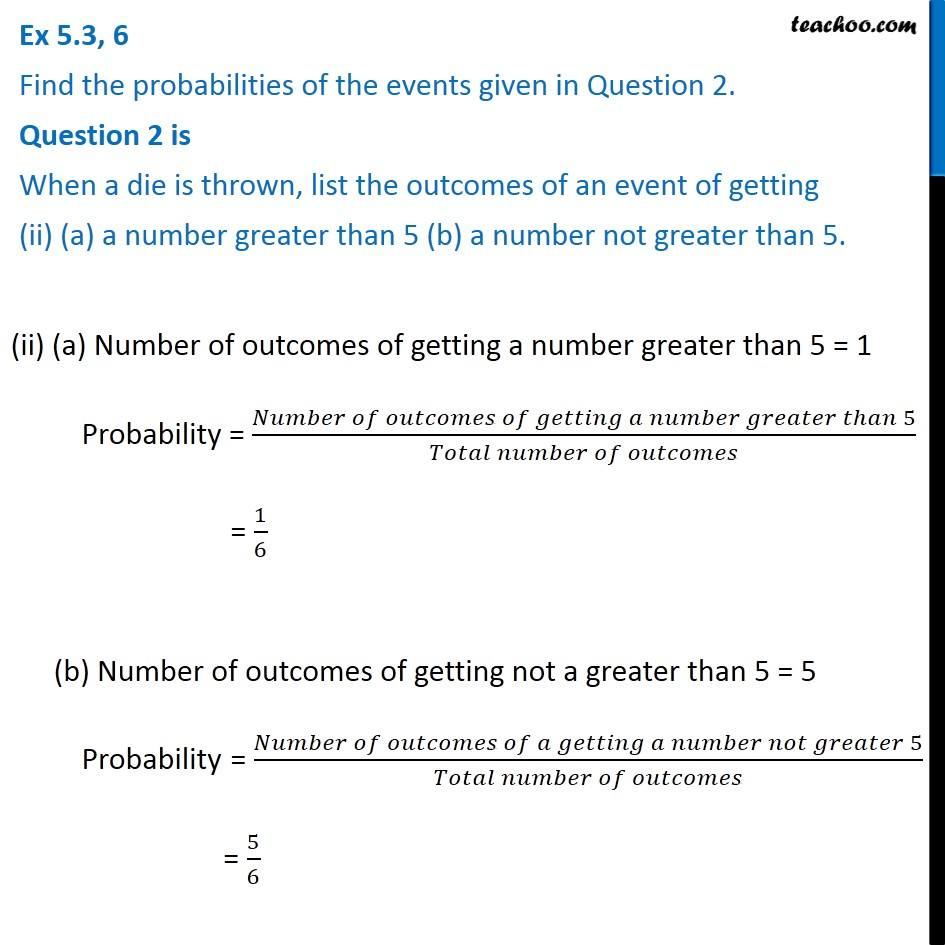Ex 4.2

Chapter 4 Class 8 Data Handling
Serial order wiseLearn in your speed, with individual attention - Teachoo Maths 1-on-1 Class

### Transcript

Ex 4.2, 6 Find the probabilities of the events given in Question 2. Question 2 is When a die is thrown, list the outcomes of an event of getting (i) (a) a prime number (b) not a prime number. Total number of outcomes = 6 (i) (a) Number of outcomes of getting a prime number = 3 Probability = (𝑁𝑢𝑚𝑏𝑒𝑟 𝑜𝑓 𝑜𝑢𝑡𝑐𝑜𝑚𝑒𝑠 𝑜𝑓 𝑔𝑒𝑡𝑡𝑖𝑛𝑔 𝑎 𝑝𝑟𝑖𝑚𝑒 𝑛𝑢𝑚𝑏𝑒𝑟)/(𝑇𝑜𝑡𝑎𝑙 𝑛𝑢𝑚𝑏𝑒𝑟 𝑜𝑓 𝑜𝑢𝑡𝑐𝑜𝑚𝑒𝑠) = 3/6=1/2 (b) Number of outcomes of getting not a prime number = 3 Probability = (𝑁𝑢𝑚𝑏𝑒𝑟 𝑜𝑓 𝑜𝑢𝑡𝑐𝑜𝑚𝑒𝑠 𝑜𝑓 𝑛𝑜𝑡 𝑔𝑒𝑡𝑡𝑖𝑛𝑔 𝑎 𝑝𝑟𝑖𝑚𝑒 𝑛𝑢𝑚𝑏𝑒𝑟)/(𝑇𝑜𝑡𝑎𝑙 𝑛𝑢𝑚𝑏𝑒𝑟 𝑜𝑓 𝑜𝑢𝑡𝑐𝑜𝑚𝑒𝑠) = 3/6=1/2 Ex 4.2, 6 Find the probabilities of the events given in Question 2. Question 2 is When a die is thrown, list the outcomes of an event of getting (ii) (a) a number greater than 5 (b) a number not greater than 5. (ii) (a) Number of outcomes of getting a number greater than 5 = 1 Probability = (𝑁𝑢𝑚𝑏𝑒𝑟 𝑜𝑓 𝑜𝑢𝑡𝑐𝑜𝑚𝑒𝑠 𝑜𝑓 𝑔𝑒𝑡𝑡𝑖𝑛𝑔 𝑎 𝑛𝑢𝑚𝑏𝑒𝑟 𝑔𝑟𝑒𝑎𝑡𝑒𝑟 𝑡ℎ𝑎𝑛 5)/(𝑇𝑜𝑡𝑎𝑙 𝑛𝑢𝑚𝑏𝑒𝑟 𝑜𝑓 𝑜𝑢𝑡𝑐𝑜𝑚𝑒𝑠) = 1/6 (b) Number of outcomes of getting not a greater than 5 = 5 Probability = (𝑁𝑢𝑚𝑏𝑒𝑟 𝑜𝑓 𝑜𝑢𝑡𝑐𝑜𝑚𝑒𝑠 𝑜𝑓 𝑎 𝑔𝑒𝑡𝑡𝑖𝑛𝑔 𝑎 𝑛𝑢𝑚𝑏𝑒𝑟 𝑛𝑜𝑡 𝑔𝑟𝑒𝑎𝑡𝑒𝑟 5)/(𝑇𝑜𝑡𝑎𝑙 𝑛𝑢𝑚𝑏𝑒𝑟 𝑜𝑓 𝑜𝑢𝑡𝑐𝑜𝑚𝑒𝑠) = 5/6